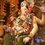# Interesting Puzzles - Cryptarithms

A cryptarithm is an arithmetic puzzle in which each digit of a fundamental operation is assigned a definite letter as in a code.

The challenge is to break the code.

These problems require skilful use of logic, & the knowledge of number theory & algebra.

The task of creating a cryptarithmic puzzle is difficult & a challenging one. As you will see in subsequent problems, the problems are skilfully designed so that actual words from the dictionary appear in the problem.

In the beginning, the task may look a challenging one, but after some practice, you will find that the solution comes naturally to you.

One reason I have not included complete steps of solving these problems is that they are too large. It takes a great amount of time & considerable space to be able to write a complete solution to cryptarithmic puzzle.

Start & try to attack these problems right on!Note by Ameya Salankar
7 years, 3 months ago

This discussion board is a place to discuss our Daily Challenges and the math and science related to those challenges. Explanations are more than just a solution — they should explain the steps and thinking strategies that you used to obtain the solution. Comments should further the discussion of math and science.

When posting on Brilliant:

• Use the emojis to react to an explanation, whether you're congratulating a job well done , or just really confused .
• Ask specific questions about the challenge or the steps in somebody's explanation. Well-posed questions can add a lot to the discussion, but posting "I don't understand!" doesn't help anyone.
• Try to contribute something new to the discussion, whether it is an extension, generalization or other idea related to the challenge.

MarkdownAppears as
*italics* or _italics_ italics
**bold** or __bold__ bold
- bulleted- list
• bulleted
• list
1. numbered2. list
1. numbered
2. list
Note: you must add a full line of space before and after lists for them to show up correctly
paragraph 1paragraph 2

paragraph 1

paragraph 2

[example link](https://brilliant.org)example link
> This is a quote
This is a quote
    # I indented these lines
# 4 spaces, and now they show
# up as a code block.

print "hello world"
# I indented these lines
# 4 spaces, and now they show
# up as a code block.

print "hello world"
MathAppears as
Remember to wrap math in $$ ... $$ or $ ... $ to ensure proper formatting.
2 \times 3 $2 \times 3$
2^{34} $2^{34}$
a_{i-1} $a_{i-1}$
\frac{2}{3} $\frac{2}{3}$
\sqrt{2} $\sqrt{2}$
\sum_{i=1}^3 $\sum_{i=1}^3$
\sin \theta $\sin \theta$
\boxed{123} $\boxed{123}$

Sort by:

Base10?

- 2 years, 10 months ago

Unless otherwise stated

- 2 years, 7 months ago

Is there any reason why the answer cannot be 42? I have 5849+0638=06487 and I'm pretty sure that checks out

- 5 years, 1 month ago

I found the rules from http://www.cryptarithms.com

• Each letter represents a unique digit.
• The solution is unique (unless otherwise stated).

So your solution does not obey the second rule. I had the exact same problem with the Send + More = Money.

- 3 years, 10 months ago

I don't think 2 different letters can be the same #

- 5 years ago

what number is repeating?

- 4 years, 11 months ago

- 5 years, 7 months ago

Well, there's just so many problems, all of the same type, Cryptorithms !

A similar problem

All have the same method, with a little bit of variation... Posting this, if somebody wants to solve problems of just 1 type, together!

- 6 years ago